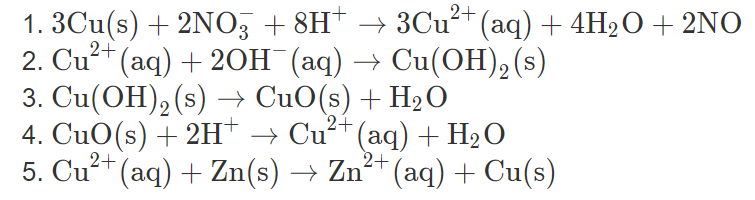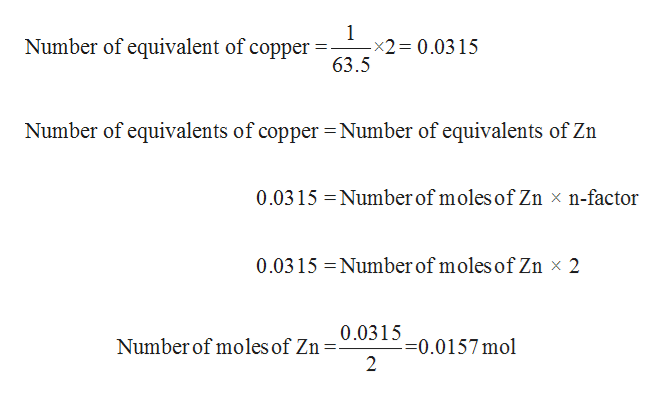# 1. ЗСu(s) + 2NO3 + 8H*2. Cur (аq) + 20Н (аq) — Сu(ОН), (s)3. Cu(ОН), (s)ЗСи* (аq) + 4Н2О + 2NO— СuО(s) + H2O(аq) + HэОZn2(aq) Cu(s)4. CuO(s)2H Cu22+5. Cu" (аq) + Zn(s

Question

27) The cycle of copper reactions illustrates important principles of chemical reactions. The cycle begins with elemental copper, which in a first reaction is oxidized to copper(II) cation. Copper(II) is then carried through different solid forms. In the last step, copper(II) cation is reduced, the copper metal is regenerated, and the cycle is closed. The five key steps are (see attached picture). Assume that you want to carry out a sequence of cycle reactions.

By how much is zinc oxidized in the fifth reaction?help_outlineImage Transcriptionclose1. ЗСu(s) + 2NO3 + 8H* 2. Cur (аq) + 20Н (аq) — Сu(ОН), (s) 3. Cu(ОН), (s) ЗСи* (аq) + 4Н2О + 2NO — СuО(s) + H2O (аq) + HэО Zn2(aq) Cu(s) 4. CuO(s)2H Cu2 2+ 5. Cu" (аq) + Zn(s fullscreen
check_circleExpert Solution
Step 1

Number of equivalents = number of moles × n- factor

Number of moles = Molarity ×Volume

Therefore,

Number of equivalents = Molarity ×Volume × n- factor

Where, n-factor is valence or charge.

The required reaction is given below.

Step 2

Here, 1 equivalent of Cu2+ is reacting with 1 equivalent of Zn. According to law of equivalents, it can be written ...help_outlineImage Transcriptionclose1 -x2 0.0315 63.5 Number of equivalent of copper Number of equivalents of copper Number of equivalents of Zn 0.0315 Number of moles of Zn x n-factor Number of moles of Zn x 2 0.0315 0.0315 =0.0157 mol 2 Number of moles of Zn fullscreen

### Want to see the full answer?

See Solution

#### Want to see this answer and more?

Solutions are written by subject experts who are available 24/7. Questions are typically answered within 1 hour*

See Solution
*Response times may vary by subject and question
Tagged in

### Other